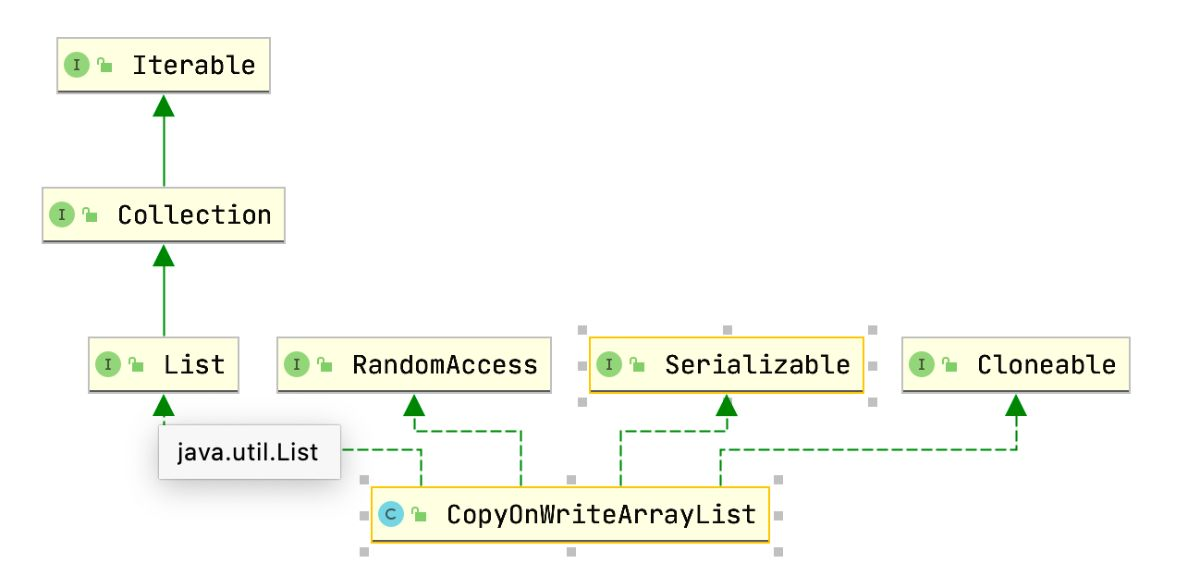# Java CopyOnWriteArrayList 源码分析## 前言

• CopyOnWriteArrayList 的实现原理，扩容机制。
• CopyOnWriteArrayList 的读写分离，弱一致性。
• CopyOnWriteArrayList 的性能如何。
• CopyOnWriteArrayList 修改元素时，为什么相同值也要重新赋值（作者 Doug Lea 这么写都是有道理的）。
• CopyOnWriteArrayList 在高版本 JDK 的实现有什么不同，为什么。## 线程安全 List

Vector 类的 get 方法：

``````// Vector 中的 get 操作添加了 synchronized
public synchronized E get(int index) {
if (index >= elementCount)
throw new ArrayIndexOutOfBoundsException(index);

return elementData(index);
}
``````

SynchronizedList 类的 ge t 方法：

``````public E get(int index) {
synchronized (mutex) {return list.get(index);}
}
``````

## CopyOnWriteArrayList

1. 并发读写时该怎么保证线程安全呢？
2. 数据要保证强一致性吗？数据读写更新后是否立刻体现？
3. 初始化和扩容时容量给多少呢？
4. 遍历时要不要保证数据的一致性呢？需要引入 Fail-Fast 机制吗？

## 分析源码 ( JDK8 )

``````/** The array, accessed only via getArray/setArray. */
private transient volatile Object[] array;
``````

CopyOnWriteArrayList 类中方法很多，这里不会一一介绍，下面会分析其中的几个常用的方法，这几个方法理解后基本就可以掌握 CopyOnWriteArrayList 的实现原理。

### 构造函数

CopyOnWriteArrayList 的构造函数一共有三个，一个是无参构造，直接初始化数组长度为0；另外两个传入一个集合或者数组作为参数，然后会把集合或者数组中的元素直接提取出来赋值给 CopyOnWriteArrayList 内部维护的数组。

``````// 直接初始化一个长度为 0 的数组
public CopyOnWriteArrayList() {
setArray(new Object);
}
// 传入一个集合，提取集合中的元素赋值到 CopyOnWriteArrayList 数组
public CopyOnWriteArrayList(Collection<? extends E> c) {
Object[] es;
if (c.getClass() == CopyOnWriteArrayList.class)
es = ((CopyOnWriteArrayList<?>)c).getArray();
else {
es = c.toArray();
if (c.getClass() != java.util.ArrayList.class)
es = Arrays.copyOf(es, es.length, Object[].class);
}
setArray(es);
}
// 传入一个数组，数组元素提取后赋值到 CopyOnWriteArrayList 数组
public CopyOnWriteArrayList(E[] toCopyIn) {
setArray(Arrays.copyOf(toCopyIn, toCopyIn.length, Object[].class));
}

``````

### 新增元素

``````/**
* Appends the specified element to the end of this list.
*
* @param e element to be appended to this list
*/
final ReentrantLock lock = this.lock;
lock.lock(); // 加锁
try {
Object[] elements = getArray(); // 获取数据数组
int len = elements.length;
Object[] newElements = Arrays.copyOf(elements, len + 1); // 拷贝一个数据数组，长度+1
newElements[len] = e; // 加入新元素
setArray(newElements); // 用新数组替换掉老数组
return true;
} finally {
lock.unlock();
}
}
``````

1. 加锁，获取目前的数据数组开始操作（加锁保证了同一时刻只有一个线程进行增加/删除/修改操作）。
2. 拷贝目前的数据数组，且长度增加一。
3. 新数组中放入新的元素。
4. 用新数组替换掉老的数组。
5. finally 释放锁。

``````public static void main(String[] args) {
CopyOnWriteArrayList<Object> copyOnWriteArrayList = new CopyOnWriteArrayList<>();
Vector vector = new Vector<>();
ArrayList arrayList = new ArrayList();

get(copyOnWriteArrayList);
get(vector);
get(arrayList);
}
public static void add(List list) {
long start = System.currentTimeMillis();
for (int i = 0; i < 100000; i++) {
}
long end = System.currentTimeMillis();
System.out.println(list.getClass().getName() + ".size=" + list.size() + ",add耗时:" + (end - start) + "ms");
}
public static void get(List list) {
long start = System.currentTimeMillis();
for (int i = 0; i < list.size(); i++) {
Object object = list.get(i);
}
long end = System.currentTimeMillis();
System.out.println(list.getClass().getName() + ".size=" + list.size() + ",get耗时:" + (end - start) + "ms");
}
``````

``````java.util.concurrent.CopyOnWriteArrayList.size=100000,add耗时:2756ms
java.util.concurrent.CopyOnWriteArrayList.size=100000,get耗时:4ms
java.util.Vector.size=100000,get耗时:5ms
java.util.ArrayList.size=100000,get耗时:2ms
``````

### 修改元素

``````public E set(int index, E element) {
final ReentrantLock lock = this.lock;
lock.lock(); //加锁
try {
Object[] elements = getArray(); // 获取老数组
E oldValue = get(elements, index); // 获取指定位置元素

if (oldValue != element) { // 新老元素是否相等，不相等
int len = elements.length;
Object[] newElements = Arrays.copyOf(elements, len); // 复制老数组
newElements[index] = element; // 指定位置赋新值
setArray(newElements); // 替换掉老数组
} else {
// Not quite a no-op; ensures volatile write semantics
setArray(elements);  // 有意思的地方来了
}
return oldValue;
} finally {
lock.unlock();
}
}
``````

``````// initial conditions
int nonVolatileField = 0;
CopyOnWriteArrayList<String> list = /* a single String */

nonVolatileField = 1;                 // (1)
list.set(0, "x");                     // (2)

String s = list.get(0);               // (3)
if (s == "x") {
int localVar = nonVolatileField;  // (4)
}
// 例子来自：https://stackoverflow.com/questions/28772539/why-setarray-method-call-required-in-copyonwritearraylist
``````

### 删除元素

`remove` 删除元素方法一共有三个，这里只看`public E remove(int index)` 方法，原理都是类似的。

``````public E remove(int index) {
final ReentrantLock lock = this.lock;
lock.lock(); // 加锁
try {
Object[] elements = getArray(); // 获取数据数组
int len = elements.length;
E oldValue = get(elements, index); // 获取要删除的元素
int numMoved = len - index - 1;
if (numMoved == 0) // 是否末尾
setArray(Arrays.copyOf(elements, len - 1)); // 数据数组减去末尾元素
else {
Object[] newElements = new Object[len - 1]; // 把要删除的数据的前后元素分别拷贝到新数组
System.arraycopy(elements, 0, newElements, 0, index);
System.arraycopy(elements, index + 1, newElements, index,
numMoved);
setArray(newElements); // 使用新数组替换老数组
}
return oldValue;
} finally {
lock.unlock(); // 解锁
}
}
``````

### 获取元素

``````public E get(int index) {
return get(getArray(), index);
}
final Object[] getArray() {
return array;
}
private E get(Object[] a, int index) {
return (E) a[index];
}
``````

1. getArray() 获取数据数组。
2. get(Object[] a, int index) 返回指定位置的元素。

### 迭代器的弱一致性

``````List<String> list = new CopyOnWriteArrayList<>();

Iterator<String> iterator = list.iterator();
while (iterator.hasNext()) {
String next = iterator.next();
System.out.println(next);
}
``````

``````www.wdbyte.com

``````

``````public Iterator<E> iterator() {
return new COWIterator<E>(getArray(), 0);
}
static final class COWIterator<E> implements ListIterator<E> {
/** Snapshot of the array */
private final Object[] snapshot;
/** Index of element to be returned by subsequent call to next.  */
private int cursor;

private COWIterator(Object[] elements, int initialCursor) {
cursor = initialCursor;
snapshot = elements;
}
......
``````

## 新版变化

``````public boolean add(E e) {
synchronized (lock) {
Object[] es = getArray();
int len = es.length;
es = Arrays.copyOf(es, len + 1);
es[len] = e;
setArray(es);
return true;
}
}
``````

``````public E set(int index, E element) {
synchronized (lock) {
Object[] es = getArray();
E oldValue = elementAt(es, index);

if (oldValue != element) {
es = es.clone();
es[index] = element;
}
// Ensure volatile write semantics even when oldvalue == element
setArray(es);
return oldValue;
}
}
``````

## 总结

1. CopyOnWriteArrayList 采用读写分离，写时复制方式实现线程安全，具有弱一致性。
2. CopyOnWriteArrayList 因为每次写入时都要扩容复制数组，写入性能不佳。
3. CopyOnWriteArrayList 在修改元素时，为了保证 volatile 语义，即使元素没有任何变化也会重新赋值，
4. 在高版 JDK 中，得益于 synchronized 锁升级策略， CopyOnWriteArrayList 的加锁方式采用了 synchronized。

1. Why setArray() method call required in CopyOnWriteArrayList.

https://stackoverflow.com/questions/28772539/why-setarray-method-call-required-in-copyonwritearraylist

1. What does volatile do?

http://www.cs.umd.edu/~pugh/java/memoryModel/jsr-133-faq.html#volatile

JDK 源码分析系列文章